# Connected component labeling – Part 4

In the previous post in this series I talked about forming a graph based on pixel neighbor relationships, and then finding the connected components of the graph. Another approach starts by finding a foreground pixel and then iteratively searching that pixel's neighbors to find the connected component. Sometimes this technique is called the flood fill approach. I'll demonstrate the basic idea using a small sample image and a series of diagrams.

The matrix below and on the left is our binary image. Using 4-connected neighbors, the image has two connected components. The matrix on the right is going to become our label matrix when we're done. It is initialized to contain all zeros.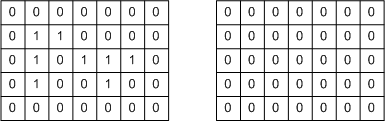Step 1: Find a foreground pixel. Set the corresponding label matrix pixel to 1, which is the first label.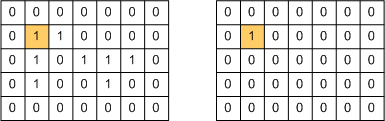Step 2: Set the pixels that were just labeled to 0, and then find all the nonzero 4-connected neighbors. Set the corresponding pixels in the label matrix to 1.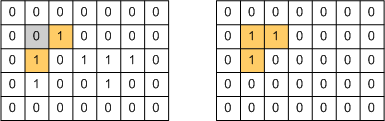Step 3: Set the pixels that were just labeled to 0, and then find all the nonzero 4-connected neighbors. Set the corresponding pixels in the label matrix to 1. (This step should seem familiar.)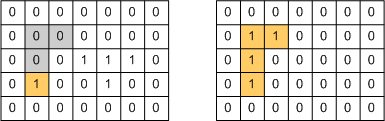Step 4: Set the pixels that were just labeled to 0, and then find all the nonzero 4-connected neighbors. Oops, there aren't any!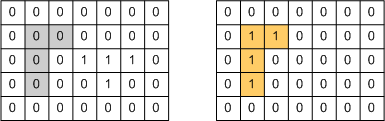Step 5: We have finished labeling object 1. Now search for the next nonzero pixel. Set the corresponding pixel in the label matrix to 2, which is the next object label.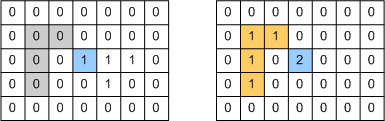Step 6: Set the pixels that were just labeled to 0, and then find all the nonzero 4-connected neighbors. Set the corresponding pixels in the label matrix to 2.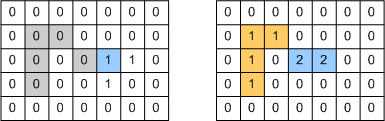Step 7: Set the pixels that were just labeled to 0, and then find all the nonzero 4-connected neighbors. Set the corresponding pixels in the label matrix to 2.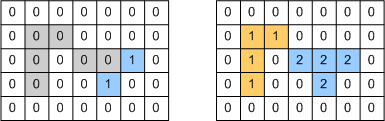Step 8: Set the pixels that were just labeled to 0, and then find all the nonzero 4-connected neighbors. Since there aren't any, we know we have finished labeling object 2. Furthermore, since there are no nonzero pixels left in the image, we know we have completed the labeling operation.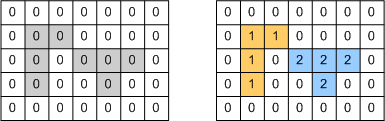Now let's talk about how you might program this in MATLAB. I'd use the neighbor indexing technique that I wrote about a couple of weeks ago. Start from this point:Call the matrix on the left bw, and call the matrix on the right L.

bw = logical([0 0 0 0 0 0 0
0 0 1 0 0 0 0
0 1 0 1 1 1 0
0 1 0 0 1 0 0
0 0 0 0 0 0 0]);

L = [0 0 0 0 0 0 0
0 1 1 0 0 0 0
0 1 0 0 0 0 0
0 0 0 0 0 0 0
0 0 0 0 0 0 0];

The linear indices of the pixels that were just labeled are 8 and 12:

idx = [8; 12];

Here are the neighbor offsets for a four-connected neighborhood:

offsets = [-1; 5; 1; -5];

"Set the pixels that were just labeled to 0".

bw(idx) = 0;

"Find all the nonzero 4-connected neighbors." Do this by adding all neighbor offsets to all pixels in the current set.

neighbors = bsxfun(@plus, idx, offsets')
neighbors =

7    13     9     3
11    17    13     7



Remove duplicates.

neighbors = unique(neighbors(:))
neighbors =

3
7
9
11
13
17



Keep only the neighbors that are nonzero.

idx = neighbors(bw(neighbors))
idx =

13



"Set the corresponding pixels in the label matrix to 1."

L(idx) = 1;

You'd repeat these operations in a loop until idx was empty, indicating that a connected component has been completely labeled.

I've covered two connected components methods in this series so far. In my next post I'll start to explain a third method - the one used by the Image Processing Toolbox functions bwlabel and bwlabeln.

PS. Good luck to everyone running in the Boston Marathon tomorrow!

Published with MATLAB® 7.4

|